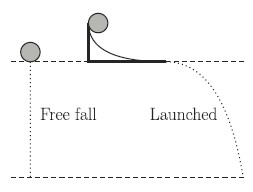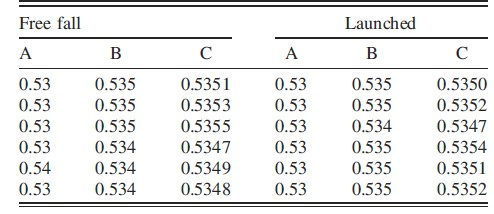# The more decimals, the more confusion

Title: Students’ conclusions from measurement data: The more decimal places, the better?
Authors: Karel Kok, Burkhard Priemer, Wiebke Musold, Amy Masnick
First author’s institution: Humboldt-Universität zu Berlin
Journal: Physical Review Physics Education Research 15, 010103 (2019)

Being able to interpret and analyze data has never been a more important skill to have, impacting people’s choices on what products and services to buy and who or what to vote for. Yet, the skill of understanding data is only taught at a basic level in most schools. Even with this instruction, prior work has found that students’ understanding of statistical concepts is overestimated and students have problems judging the quality of data. Further many students are able to perform the calculations needed to understand the data but do not understand what those calculations mean.

Typically lacking in any statistics instruction is measurement uncertainty and sources of variance in data, both of which are central to understanding data. For example, a measurement can only be as good as the tool used to make the measurement and multiple samples can give a range of means. Generally, students only have an intuitive knowledge about uncertainty: the more exact the data, the better the quality. The goal of today’s paper is to see how students respond to data that seems to contradict this intuitive idea, namely, more precise data but more variance. Specifically, the authors of today’s paper investigated how increasing the number of decimal places shown in a data table would affect how students reasoned about the outcome of an experiment.

First some context: the authors selected 153 students in grades 8-10 at an urban German high school for this study, which they conducted during a normal 45 minute class period. The class began with the researchers showing a video clip explaining an experiment: a ball is dropped from rest at some height. A second ball is then rolled down the ramp and rolls horizontally before falling from the same height as the first ball (figure 1).Figure 1: A sketch of the experimental setup in the video. As both balls are dropped from the same height, they will have the same falling time. Fig 1 in paper.

The students were then randomly assigned to group A, B, or C and asked to fill out the corresponding questionnaire. The questionnaire first asked the student to predict which ball had the longest falling time (the ball that started at rest, the ball that was rolled, or both times are equal). After making a prediction, the students were given the data the experimenter collected during the video, which is shown in figure 2.Figure 2: Students in group A were shown the data in the two columns labeled “A”, students in group B were shown the data in the two columns labeled “B”, and so on. Notice that there is a varying number of decimal places in each column. (Table 1 in paper)

Students who were in group A saw a data table where all the values had 2 decimal places, students in group B saw a data table where all the values had 3 decimal places, and students in group C saw a data table where all the values had 4 decimal places. Students then answered a multiple choice question about how they would analyze the data and whether the data showed a “clear difference,” “small difference,” or “no difference” between the two experimental conditions. After examining the data, the student was asked to reevaluate their prediction and explain their reasoning for which ball had a longer fall time. Initially, half of the students thought that the rolled ball would have the longest drop time, a third thought both balls would take the same time to fall, and the remaining students thought the ball that started at rest would have the longest drop time.

So what did the researchers find? First the researchers classified students according to their initial hypothesis and their final hypothesis. The researchers found that 40% of the students in group A (the two decimal group) switched from an incorrect prediction to a correct prediction while 31% and 33% of the students in groups B and C respectively switched from an incorrect prediction to a correct prediction. However, while none of the students in group A switched from the correct hypothesis to an incorrect hypothesis, around 10% of the participants in each of groups B and C switched from a correct hypothesis to an incorrect hypothesis. In each group, most of the students stated that they analyzed the data by finding the sum of the rows of data or the mean of the data for each experimental condition and that most students reported noticing small differences between the data for each condition.

Next the researchers classified the explanations of the reevaluated hypotheses to understand why students who saw data with more decimal places were more likely to change their correct hypothesis to an incorrect one. Across all 3 groups, 32 students used quantitative reasoning while 112 students used qualitative reasoning; however, students in group A were twice as likely to use quantitative reasoning as students in groups B and C. Those students who did use quantitative reasoning picked the correct hypothesis when reevaluating their hypothesis 84% of the time while those who used qualitative reasoning picked the correct hypothesis when reevaluating their hypothesis 54% of the time.

After finding these results, the researchers attempted to explain why these results were observed. First, as the students were in high school, they had not been taught how to judge the size of a mean difference and if it is significant. Therefore, if the student found that the difference between the means of the two conditions was not exactly zero, the student may have thought that one of the conditions must have had a longer fall time.

Second, the more exact the data was, the more likely the student was to use qualitative reasoning rather than quantitative reasoning. As the data provided were quantitative, this suggests that students who saw more exact data tended to disregard it and instead reason based on intuition. Since intuition would suggest that the rolled ball would take longer to fall, the students who based their reasoning on intuition would not choose the correct hypothesis.

So what can we take away from this paper? First, increasing the number of decimals seems to reduce high school students’ ability to compare data sets. However, the remedy isn’t just to decrease the number of decimals used in class because real data isn’t without uncertainty or set to a fixed number of decimal places. Instead, instruction should talk about variance and confidence intervals instead of just about means. Further, when assessing labs and calculations, the variance and “spread” of the data should be treated equally with the “answer” or value that was calculated. Finally, as data analysis is a skill that extends beyond science, these discussions of variance and quality of data should appear in all subjects.